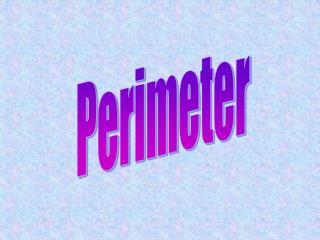DownloadDownload PresentationPerimeter

# Perimeter

Télécharger la présentation## Perimeter

- - - - - - - - - - - - - - - - - - - - - - - - - - - E N D - - - - - - - - - - - - - - - - - - - - - - - - - - -
##### Presentation Transcript

1. Perimeter

2. Perimeter- the distance around a figure You can find the perimeterof any polygon by adding the lengths of all its sides. 4 cm 4 cm 6 cm 4 cm + 4 cm + 6 cm = 14 cm Perimeter = 14 cm

3. Find the perimeter. 1 cm 10 cm 4 cm 3 cm 4 cm 10 cm 1 cm 3 cm + 10 cm + 1 cm + 4 cm + 4 cm + 1 cm + 10 cm = 33 cm Perimeter = 33 cm

4. You can find the perimeterof a regular polygon by multiplying the length of one side by the number of sides. 6 cm 7 cm 7 cm x 6 = 42 cm 6 cm x 5 = 30 cm Perimeter= 30 cm Perimeter= 42 cm

5. Remember: a regular polygon has equal sides and equal angles 8 cm 12 mm 8 cm x 4 = 32 cm 12 mm x 3 = 36 mm Perimeter = 32 mm Perimeter = 36 mm

6. The perimeter(P) of a rectangle equals 2 times the sum of the length (l) and the width (w). 10 cm P = 2 x (l + w) 5 cm P = 2 x (10 + 5) P = 2 x 15 l =10 cm w = 5 cm P = 30 cm

7. Area

8. Area- measure of the surface of a region in square units. Examples of Units Square Units mm mm x mm = mm2 cm cm x cm = cm2 m m x m = m2 in in x in = in2 ft ft x ft = ft2 yd yd x yd = yd2

9. You can use this formula to find the area of a rectangle. Area = length x width A = l x w 5 cm width (w) A = 10 cm x 5 cm 10 cm length (l) A = 50 cm2 The area is 50 cm2.

10. There is a special formula for the area of a square. (s = side) A = s x s = s2 5 cm A = s2 5 cm A = 5 cm x 5 cm = 52 cm2 A = 25 cm2 The area is 25 cm2.

11. height (h) 4 in base (b) 6 in You can use the base and height to find the area of a right triangle. Math Tip: The height is always perpendicular to the base. A = 1/2 x (b x h) A = 1/2 x (6 x 4) A = 1/2 x (24) A = 12 in2 The area is 12 in2. Remember: solve inside the parenthesis first

12. You can use the formulaA = 1/2 x (b x h) to find the area of any triangle. Math Tip: The height is always perpendicular to the base. A = 1/2 x (b x h) height (h) 10 cm A = 1/2 x (4 x 10) A = 1/2 x (40) A = 20 cm2 The area is 20 cm2. base (b) 4 cm

13. You can use a formula to find the area of any parallelogram. D A 12 ft 12 ft A D height (h) 5 ft 5 ft B B C base (b) 12 ft M P C You can use this formula to find the area of a parallelogram: ABP to DCM Slide Area of rectangleAPMD: A = b x h A = 12 ft x 5 ft A = 12 ft x 5 ft A = 60 ft2 A = 60 ft2